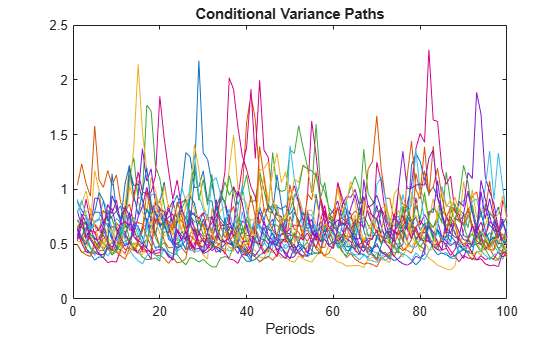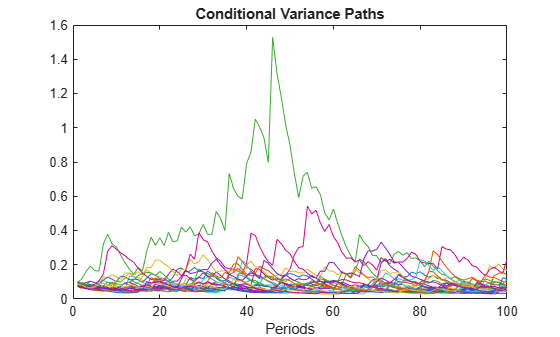Documentation

# filter

Filter disturbances through conditional variance model

## Syntax

``````[V,Y] = filter(Mdl,Z)``````
``````[V,Y] = filter(Mdl,Z,Name,Value)``````

## Description

example

``````[V,Y] = filter(Mdl,Z)``` filters disturbances (`Z`) through the fully specified conditional variance model (`Mdl`) to produce conditional variances (`v`) and responses (`y`). `Mdl` can be a `garch`, `egarch`, or `gjr` model.```

example

``````[V,Y] = filter(Mdl,Z,Name,Value)``` filters disturbances using additional options specified by one or more `Name,Value` pair arguments. For example, you can specify presample disturbance and conditional variance paths.```

## Examples

collapse all

Specify a GARCH(1,1) model with Gaussian innovations.

`Mdl = garch('Constant',0.005,'GARCH',0.8,'ARCH',0.1);`

Simulate the model using Monte Carlo simulation. Then, standardize the simulated innovations and filter them.

```rng(1); % For reproducibility [v,e] = simulate(Mdl,100,'E0',0,'V0',0.05); Z = e./sqrt(v); [V,E] = filter(Mdl,Z,'Z0',0,'V0',0.05);```

Confirm that the outputs of `simulate` and `filter` are identical.

`isequal(v,V)`
```ans = logical 1 ```

The logical value `1` confirms that the two outputs are identical.

Specify an EGARCH(1,1) model with Gaussian innovations.

```Mdl = egarch('Constant',-0.1,'GARCH',0.8,'ARCH',0.3,... 'Leverage',-0.05);```

Simulate 25 series of standard Gaussian observations for 100 periods.

```rng(1); % For reproducibility Z = randn(100,25);```

`Z` represents 25 paths of synchronized disturbances for 100 periods.

Obtain 25 paths of conditional variances by filtering the disturbance paths through the EGARCH(1,1) model.

`V = filter(Mdl,Z);`

Plot the paths of conditional variances.

```figure; plot(V); title('Conditional Variance Paths'); xlabel('Periods');```Specify a GJR(1,2) model with Gaussian innovations.

```Mdl = gjr('Constant',0.005,'GARCH',0.8,'ARCH',{0.1 0.01},... 'Leverage',{0.05 0.01});```

Simulate 25 series of standard Gaussian observations for 102 periods.

```rng(1); % For reproducibility Z = randn(102,25);```

`Z` represents 25 paths of synchronized disturbances for 102 periods.

Obtain 25, 100 period paths of conditional variances by filtering the disturbance paths through the GJR(1,2) model. Specify the first two disturbances as presample observations.

`V = filter(Mdl,Z(3:end,:),'Z0',Z(1:2,:));`

Plot the paths of conditional variances.

```figure; plot(V); title('Conditional Variance Paths'); xlabel('Periods');```## Input Arguments

collapse all

Conditional variance model without any unknown parameters, specified as a `garch`, `egarch`, or `gjr` model object.

`Mdl` cannot contain any properties that have `NaN` value.

Disturbance paths used to drive the innovation process, specified as a numeric vector or matrix. Given the variance process, ${\sigma }_{t}^{2},$ and the disturbance process zt, the innovation process is

`${\epsilon }_{t}={\sigma }_{t}{z}_{t}.$`

As a column vector, `Z` represents a single path of the underlying disturbance series.

As a matrix, the rows of `Z` correspond to periods. The columns correspond to separate paths. The observations across any row occur simultaneously.

The last element or row of `Z` contains the latest observation.

### Note

`NaN`s indicate missing values. `filter` removes these values from `Z` by listwise deletion. The software removes any row of `Z` with at least one `NaN`. Removing `NaN`s in the data reduces the sample size, and can also create irregular time series.

### Name-Value Pair Arguments

Specify optional comma-separated pairs of `Name,Value` arguments. `Name` is the argument name and `Value` is the corresponding value. `Name` must appear inside quotes. You can specify several name and value pair arguments in any order as `Name1,Value1,...,NameN,ValueN`.

Example: `'Z0',[1 1;0.5 0.5],'V0',[1 0.5;1 0.5]` specifies two equivalent presample paths of innovations and two, different presample paths of conditional variances.

Presample disturbance paths, specified as a numeric vector or matrix. `Z0` provides initial values for the input disturbance series, `Z`.

• If `Z0` is a column vector, then `filter` applies it to each output path.

• If `Z0` is a matrix, then it must have at least as many columns as `Z`. If `Z0` has more columns than `Z`, then `filter` uses the first `size(Z,2)` columns only.

`Z0` must have at least `Mdl.Q` rows to initialize the conditional variance model. If the number of rows in `Z0` exceeds `Mdl.Q`, then `filter` uses the latest required number of observations only.

The last element or row contains the latest observation.

By default, `filter` sets any necessary presample disturbances to an independent sequence of standardized disturbances drawn from `Mdl.Distribution`.

Data Types: `double`

Positive presample conditional variance paths, specified as a numeric vector or matrix. `V0` provides initial values for the conditional variances in the model.

• If `V0` is a column vector, then `filter` applies it to each output path.

• If `V0` is a matrix, then it must have at least as many columns as `Z`. If `V0` has more columns than `Z`, then `filter` uses the first `size(Z,2)` columns only.

`V0` must have at least `max(Mdl.P,Mdl.Q)` rows to initialize the variance equation. If the number of rows in `V0` exceeds the necessary number, then `filter` uses the latest required number of observations only.

The last element or row contains the latest observation.

By default, `filter` sets any necessary presample conditional variances to the unconditional variance of the process.

Data Types: `double`

### Notes

• `NaN`s indicate missing values. `filter` removes missing values. The software merges the presample data (`Z0` and `V0`) separately from the disturbances (`Z`), and then uses list-wise deletion to remove rows containing at least one `NaN`. Removing `NaN`s in the data reduces the sample size. Removing `NaN`s can also create irregular time series.

• `filter` assumes that you synchronize presample data such that the latest observation of each presample series occurs simultaneously.

## Output Arguments

collapse all

Conditional variance paths, returned as a column vector or matrix. `V` represents the conditional variances of the mean-zero, heteroscedastic innovations associated with `Y`.

The dimensions of `V` and `Z` are equivalent. If `Z` is a matrix, then the columns of `V` are the filtered conditional variance paths corresponding to the columns of `Z`.

Rows of `V` are periods corresponding to the periodicity of `Z`.

Response paths, returned as a numeric column vector or matrix. `Y` usually represents a mean-zero, heteroscedastic time series of innovations with conditional variances given in `V`.

`Y` can also represent a time series of mean-zero, heteroscedastic innovations plus an offset. If `Mdl` includes an offset, then `filter` adds the offset to the underlying mean-zero, heteroscedastic innovations. Therefore, `Y` represents a time series of offset-adjusted innovations.

If `Z` is a matrix, then the columns of `Y` are the filtered response paths corresponding to the columns of `Z`.

Rows of `Y` are periods corresponding to the periodicity of `Z`.

## Alternatives

`filter` generalizes `simulate`. Both function filter a series of disturbances to produce output responses and conditional variances. However, `simulate` autogenerates a series of mean-zero, unit-variance, independent and identically distributed (iid) disturbances according to the distribution in the conditional variance model object, `Mdl`. In contrast, `filter` lets you directly specify your own disturbances.

 Bollerslev, T. “Generalized Autoregressive Conditional Heteroskedasticity.” Journal of Econometrics. Vol. 31, 1986, pp. 307–327.

 Bollerslev, T. “A Conditionally Heteroskedastic Time Series Model for Speculative Prices and Rates of Return.” The Review of Economics and Statistics. Vol. 69, 1987, pp. 542–547.

 Box, G. E. P., G. M. Jenkins, and G. C. Reinsel. Time Series Analysis: Forecasting and Control. 3rd ed. Englewood Cliffs, NJ: Prentice Hall, 1994.

 Enders, W. Applied Econometric Time Series. Hoboken, NJ: John Wiley & Sons, 1995.

 Engle, R. F. “Autoregressive Conditional Heteroskedasticity with Estimates of the Variance of United Kingdom Inflation.” Econometrica. Vol. 50, 1982, pp. 987–1007.

 Hamilton, J. D. Time Series Analysis. Princeton, NJ: Princeton University Press, 1994.